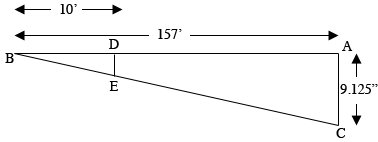SEARCH HOMEMath Central Quandaries & QueriesQuestion from Mark: I'm trying to calculate slope over 157'.I have a drop of 9 1/8 inches. What fraction of an inch of fall do I have every 10 feetHi Mark,

Here is my diagram, not to scale.Triangles $ABC$ and $DBE$ are similar.What is the length of the line segment $DE?$

PennyMath Central is supported by the University of Regina and The Pacific Institute for the Mathematical Sciences.